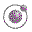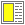The Order of Evaluation - slide 12 : 26

The eta rewrite rule
An eta conversion lifts certain function calls out of a lambda convolute

A function f, which only passes its parameters on to another function e, can be substituted by e

(lambda(x) (e x)) <=> e   provided that x is not free in the expression e
Legal conversion:
 Expression Converted Expression `(lambda (x) (square x))` `square`
Illegal conversion:
 Expression Converted Expression `(lambda(x) ((lambda(y) (f x y)) x))` `(lambda(y) (f x y))`